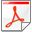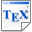[BBC+11] T. Brázdil, V. Brožek, K. Chatterjee, V. Forejt and A. Kučera. Two Views on Multiple Mean-Payoff Objectives in Markov Decision Processes. In Proc. 26th Annual IEEE Symposium on Logic in Computer Science (LICS'11), pages 33-42, IEEE Computer Society. June 2011. [pdf] [bib] Downloads:pdf (367 KB)bib Notes: Please see [BBC+14] for a journal version of this paper. Links: [Google] [Google Scholar] [CiteSeer] Abstract. We study Markov decision processes (MDPs) with multiple limit-average (or mean-payoff) functions. We consider two different objectives, namely, expectation and satisfaction objectives. Given an MDP with k reward functions, in the expectation objective the goal is to maximize the expected value, and in the satisfaction objective the goal is to maximize the probability of runs such that the limit-average value stays above a given vector. We show that under the expectation objective, in contrast to the single-objective case, both randomization and memory are necessary for strategies, and we show that finite-memory randomized strategies are sufficient. We show that under the satisfaction objective, in contrast to the single-objective case, randomization is necessary for strategies, and we show that randomized memoryless strategies are sufficient for epsilon-approximation, for all epsilon>0. We show that the decision problems for both expectation and satisfaction objectives can be solved in polynomial time and the trade-off curve (Pareto curve) can be epsilon-approximated in time polynomial in the size of the MDP and 1/epsilon, and exponential in the number of reward functions, for all epsilon>0. Our results also reveal flaws in an earlier paper ("Markov Decision Processes with multiple Long-Run Average Objectives", FSTTCS 2007) for MDPs with multiple mean-payoff functions under the expectation objective, correct the flaws and obtain improved results.